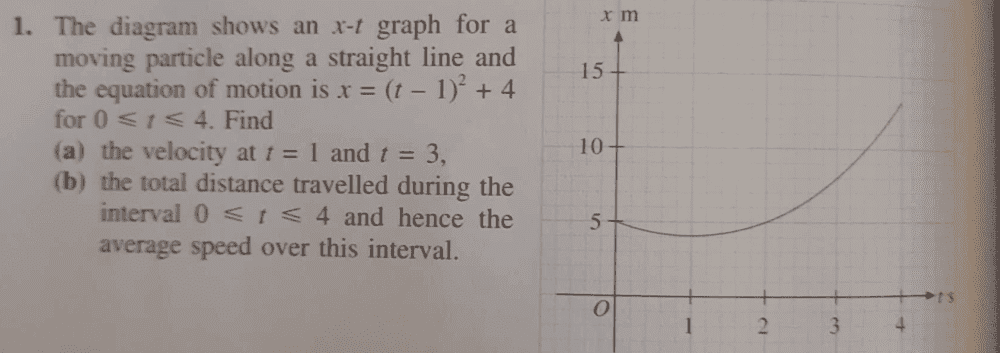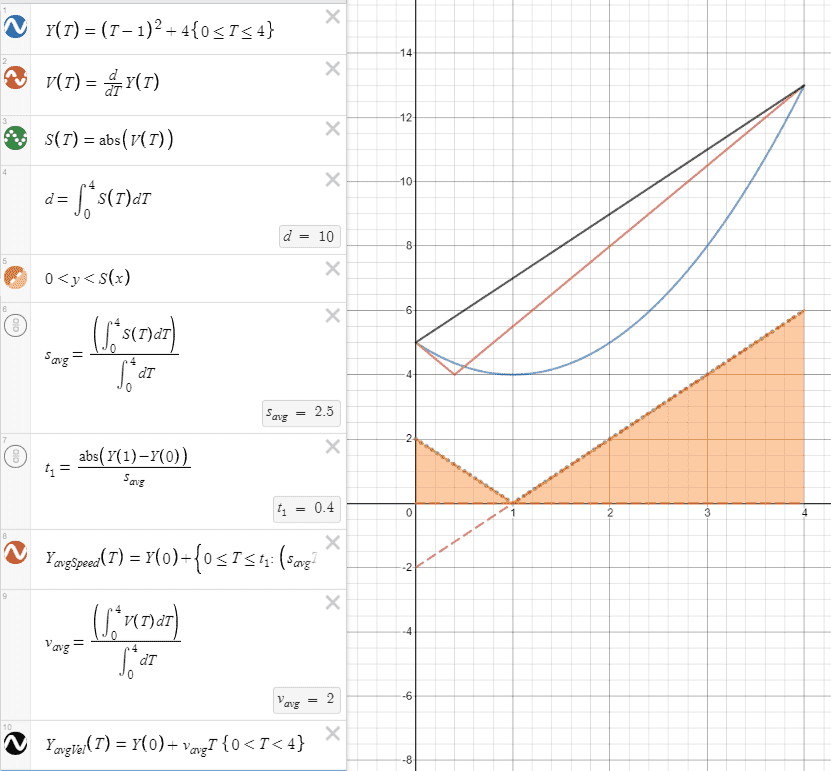# Finding distance on a distance-time graph

• chwala
In summary, the conversation discusses a question about calculating distance and average speed. The answer provided explains the concept of average speed and provides two different approaches for solving the problem. The conversation also includes a discussion about the difference between average speed and average velocity.

#### chwala

Gold Member
Homework Statement
find the distance travelled by considering the distance-time graph below
Relevant Equations
travel graphsthis question caught me by surprise, i had made an assumption that its straight forward...my interest is on question 1.b

the total distance traveled is ##1+1+8=10##...
average speed is therefore = ##2.5m/s##

Hello !

chwala said:
my interest is on question 1.b

BvU said:
Hello !

i do not really have a question, i am looking for an alternative approach...or can i assume there isn't?

chwala said:
i do not really have a question, i am looking for an alternative approach...or can i assume there isn't?
Right, there isn't. Just look at the graph to see how the position changes each second. Note that although the particle traveled 10 units, its displacement changed by only 8 units. In the first second it moved backward by 1 unit, then forward in the second second to get to its original position, then moved 8 units in the next two seconds, from 5 to 13. So although the distance traveled was 1 + 1 + 8 = 10 units, its change in position was -1 + 1 + 8 = 8 units.

•chwala
Mark44 said:
Right, there isn't. Just look at the graph to see how the position changes each second. Note that although the particle traveled 10 units, its displacement changed by only 8 units. In the first second it moved backward by 1 unit, then forward in the second second to get to its original position, then moved 8 units in the next two seconds, from 5 to 13. So although the distance traveled was 1 + 1 + 8 = 10 units, its change in position was -1 + 1 + 8 = 8 units.
Thanks mark, you've given me help for the last 10 years...bingo from Africa!

•Mark44
chwala said:
Thanks mark, you've given me help for the last 10 years...bingo from Africa!
I knew it had be a while, but didn't realize that it had been 10 years.

chwala said:
i do not really have a question, i am looking for an alternative approach...or can i assume there isn't?

While your graphical approach works great,
here's an approach that relies on the calculus definitions [since this is a calculus and beyond subforum]
and gives an interpretation.
(Screencaptured from a desmos calculation. Type it in yourself at https://www.desmos.com/calculator .)The $Y_{avgSpeed}$ line is a little long:
$Y_{avgSpeed}\left(T\right)=Y\left(0\right)+\left\{0\le T\le t_{1}:\ \left(s_{avg}T\cdot\operatorname{sign}\left(Y\left(1\right)-Y\left(0\right)\right)\right)\ ,t_{1}\le T\le4:\ \left(\left(Y\left(1\right)-Y\left(0\right)\right)+s_{avg}\left(T-t_{1}\right)\cdot\operatorname{sign}\left(Y\left(4\right)-Y\left(1\right)\right)\right)\right\}$
(You can right-click on the equation, Show Math as $\TeX$ commands, then copy to the clipboard, then paste into a Desmos cell.)

• Speed $S$ is the magnitude of the [instantaneous] velocity $V$.
• Distance $d$ traveled is the integral of the speed function over time.
• Average-speed $s_{avg}$ is the time-weighted average of speed,
and is equal to the total distance divided by the total time.
The average-speed is
the constant speed of a traveler to travel the same total distance along the same path in space in the same time
as the given variable-velocity path.

This is, of course, different from the average-velocity,
which is the
the constant velocity of a traveler to travel the same total displacement between the endpoints in space in the same time
as the given variable-velocity path.

•chwala
robphy said:
While your graphical approach works great,
here's an approach that relies on the calculus definitions [since this is a calculus and beyond subforum]
and gives an interpretation.
(Screencaptured from a desmos calculation. Type it in yourself at https://www.desmos.com/calculator .)

View attachment 278211
The $Y_{avgSpeed}$ line is a little long:
$Y_{avgSpeed}\left(T\right)=Y\left(0\right)+\left\{0\le T\le t_{1}:\ \left(s_{avg}T\cdot\operatorname{sign}\left(Y\left(1\right)-Y\left(0\right)\right)\right)\ ,t_{1}\le T\le4:\ \left(\left(Y\left(1\right)-Y\left(0\right)\right)+s_{avg}\left(T-t_{1}\right)\cdot\operatorname{sign}\left(Y\left(4\right)-Y\left(1\right)\right)\right)\right\}$
(You can right-click on the equation, Show Math as $\TeX$ commands, then copy to the clipboard, then paste into a Desmos cell.)

• Speed $S$ is the magnitude of the [instantaneous] velocity $V$.
• Distance $d$ traveled is the integral of the speed function over time.
• Average-speed $s_{avg}$ is the time-weighted average of speed,
and is equal to the total distance divided by the total time.
The average-speed is
the constant speed of a traveler to travel the same total distance along the same path in space in the same time
as the given variable-velocity path.

This is, of course, different from the average-velocity,
which is the
the constant velocity of a traveler to travel the same total displacement between the endpoints in space in the same time
as the given variable-velocity path.
impressive boss!